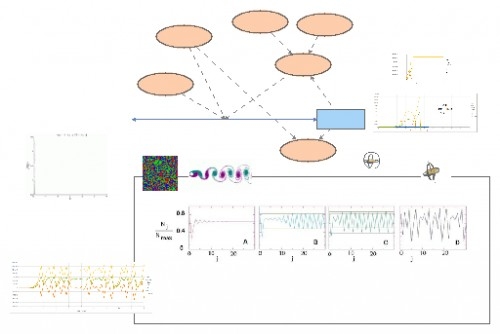Clone of POPULATION LOGISTIC MAP (WITH FEEDBACK)The simulation integrates or sums (INTEG) the Nj population, with a change of Delta N in each generation, starting with an initial value of 5.
The equation for DeltaN is a version of
Nj+1 = Nj  + mu (1- Nj / Nmax ) Nj
the maximum population is set to be one million, and the growth rate constant mu = 3.

Nj: is the “number of items” in our current generation.

Delta Nj: is the “change in number of items” as we go from the present generation into the next generation. This is just the number of items born minus the number of items who have died.

mu: is the growth or birth rate parameter, similar to that in the exponential growth and decay model. However, as we extend our model it will no longer be the actual growth rate, but rather just a constant that tends to control the actual growth rate without being directly proportional to it.

F(Nj) = mu(1‐Nj/Nmax): is our model for the effective “growth rate”, a rate that decreases as the number of items approaches the maximum allowed by external factors such as food supply, disease or predation. (You can think of mu as the growth or birth rate in the absence of population pressure from other items.) We write this rate as F(Nj), which is a mathematical way of saying F is affected by the number of items, i.e., “F is a function of Nj”. It combines both growth and all the various environmental constraints on growth into a single function. This is a good approach to modeling; start with something that works (exponential growth) and then modify it incrementally, while still incorporating the working model.

Nj+1 = Nj + Delta Nj : This is a mathematical way to say, “The new number of items equals the old number of items plus the change in number of items”.

Nj/Nmax: is what fraction a population has reached of the maximum "carrying capacity" allowed by the external environment. We use this fraction to change the overall growth rate of the population. In the real world, as well as in our model, it is possible for a population to be greater than the maximum population (which is usually an average of many years), at least for a short period of time. This means that we can expect fluctuations in which Nj/Nmax is greater than 1.

This equation is a form of what is known as the logistic map or equation. It is a map because it "maps'' the population in one year into the population of the next year. It is "logistic'' in the military sense of supplying a population with its needs. It a nonlinear equation because it contains a term proportional to Nj^2 and not just Nj. The logistic map equation is also an example of discrete mathematics. It is discrete because the time variable j assumes just integer values, and consequently the variables Nj+1 and Nj do not change continuously into each other, as would a function N(t). In addition to the variables Nj and j, the equation also contains the two parameters mu, the growth rate, and Nmax, the maximum population. You can think of these as "constants'' whose values are determined from external sources and remain fixed as one year of items gets mapped into the next year. However, as part of viewing the computer as a laboratory in which to experiment, and as part of the scientific process, you should vary the parameters in order to explore how the model reacts to changes in them.